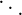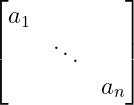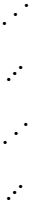# How to denote diagonal dots symbol in LaTeX?

Latex diagonal dots are the sum of three dots located along the diagonal. This diagonal dots symbol is represented by the \ddots command.

 Properties Value Symbol Diagonal dots Package Default Command \ddots Argument No Example \ddots →These diagonal dots are used to represent a matrix with n number of elements.

\documentclass{article}
\begin{document}
$\ddots$
\end{document}

Output :And you see the diagonal matrix where the elements of the matrix are arranged just along the diagonal. In that case, only the latex diagonal dots symbol is used.

\documentclass{article}
\usepackage{amsmath}
\begin{document}
$\begin{bmatrix} a_{1} & & \\ & \ddots & \\ & & a_{n} \end{bmatrix}$
\end{document}

Output :## Inverse diagonal dots

Inverse diagonal dots are located in a diagonal direction from south-west to north-east.

Latex has more than one command and method to represent this inverse diagonal dots symbol.

 Package command MySymbol \udots Mathdots \iddots yhmath \adots graphicx \reflectbox{...}
\documentclass{article}
\usepackage{graphicx}
\usepackage{yhmath}
\usepackage{mathdots}
\usepackage{MnSymbol}
\begin{document}
$\iddots$
$\udots$
$\adots$
$\reflectbox{\ddots}$
\end{document}

Output :$\ddots$ is passed as an argument in \reflectbox command which converts diagonal dots symbol to inverse diagonal dots symbol. And for this you need to take the help of graphicx package.#### Md Jidan Mondal

LaTeX expert with over 10 years of experience in document preparation and typesetting. Specializes in creating professional documents, reports, and presentations using LaTeX.